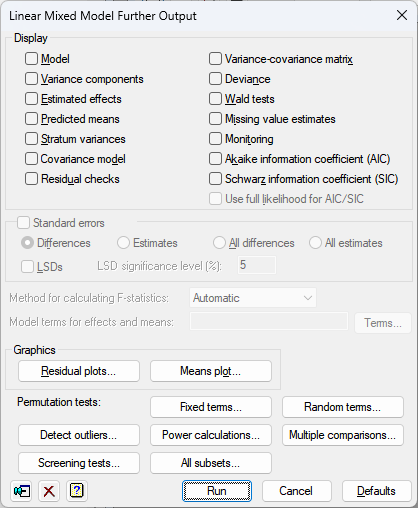1. Home
2. Linear Mixed Model Further Output

# Linear Mixed Model Further Output

Use this to select further output following any Linear Mixed Model (REML) analysis.
Although the dialog is labelled by the menu using it, they all use the same dialog
and this help page covers all the options available in any of the REML menus.
In some analyses, the some of the options will not be applicable and will
not show on the dialog (e.g. Detect outliers is only shown for menus with models which have no spline or covariance models).## Display

This specifies which items of output are to be produced by the analysis.

 Model Description of the model fitted by the analysis Variance components Estimates of variance parameters Estimated effects Estimates of regression coefficients Predicted means Predicted means Residual checks Uses the VCHECK procedure to check the residuals for outliers and variance stability Stratum Variances Estimates of approximate stratum variances Variance-covariance matrix Variance-covariance matrix for the variance parameters Deviance The residual deviance Wald tests Wald Tests for fixed model terms and accompanying F-statistics Covariance model Estimated covariance models in matrix format Residual checks Uses the VCHECK procedure to check the residuals for outliers and variance stability Monitoring Monitoring information at each iteration Akaike information coefficient (AIC) Akaike information coefficient to assess the random model Schwarz information coefficient (SIC) Schwarz information coefficient to assess the random model Use full likelihood for AIC/SIC The information coefficients are calculated using the full log-likelihood from the VKEEP procedure, otherwise the residual log-likelihood is used. See the VAIC procedure for details.

## Standard errors

Tables of means and effects are accompanied by estimates of standard errors. You can choose whether Genstat computes standard errors or standard errors of differences (SEDs) for the tables. Approximate least significant differences (LSDs) for the predicted means of the fixed terms specified in the Model terms for effects and means field can be computed by selecting LSDs. These are calculated using the approximate numbers of residual degrees of freedom printed by the analysis in the d.d.f column in the table of tests for fixed tests (produced by selecting the Wald tests display option). The LSDs will only be produced if the Predicted means option has been selected. The degrees of freedom are relevant for assessing the fixed term as a whole, and may vary over the contrasts amongst the means of the term. So the LSDs should be used with caution. If you are interested in a specific comparison, you should set up a 2-level factor to fit this explicitly in the analysis. The significance level for LSDs can be specified as a percentage (default 5) in the accompanying field.

## Method for calculating F-statistics

This controls whether Wald tests for fixed effects are accompanied with approximate F statistics and corresponding numbers of residual degrees of freedom. The computations, using the method devised by Kenward & Roger (1997), can be time consuming with large or complicated models. So, the default setting automatic, can be used to allow Genstat to assess the model itself and decide automatically whether to do the computations and which method to use. The other settings allow you to control what to do yourself:

 none No F statistics are produced algebraic F statistics are calculated using algebraic derivatives (which may involve large matrix calculations) numerical F statistics are calculated using numerical derivatives (which require an extra evaluation of the mixed model equations for every variance parameter).

## Model terms for effects and means

This specifies the model terms, as a formula, for which tables of means and/or effects are displayed. For covariates, the associated linear regression parameter can be printed as an effect, but predicted means are not available. Predicted means for other terms are adjusted to the mean of the covariate (but see note below). The formula can include the string ‘Constant‘ to include entries for the constant term.

If no formula is specified, means or effects are produced for all the fixed model terms and none of the random terms.

## Graphics

The residual plots button can be used to generate plots of residuals. For the linear mixed models and spatial models – regular grid menus an extended residual plot dialog is opened where you can plot residuals in field layout. For the spatial models – regular grid menu the Display variogram button can be used to display the residuals in a variogram.

## Detect outliers

The Detect Outliers button can be used to run a model to detect outliers.

## Power calculations

The Power Calculations button can be used to calculate the power for a fixed model term or contrast in the analysis.

## Permutation test

The Permutation Test button can be used to determine the significance of a random term in the model using a permutation test.

## Screening tests

The Screening tests button can be used to determine the significance of the fixed terms in the model by calculating marginal and conditional tests for these.

## All subsets

The All subsets button can be used to fit all subsets of the Fixed model and select the best of these.

## Multiple comparisons

This button will open the Multiple Comparisons for REML menu which lets you request output of multiple comparison tests.

This option is only available if the option Show multiple comparisons on menus has been enabled on the Menus tab available from the Tools | Options command on the menu bar.